# Some extra problems for MAT 200

1. Write the negation of each of the following statements (in English, not symbolically).
1. If it rains, then either I will wear a coat or I'll stay home.
2. This function has no inverse, and it is not continuous.
3. In any triangle, the sum of the measure of the angles is less than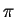.
4. For every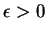, there is aso thatwhenever.
5. Every natural number has a unique additive inverse.

2. Prove or disprove each of the following statements, using only the axioms in Appendix 1. Define the set of integers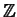by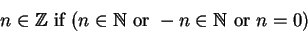As usual, we say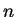is negative if, andis positive if.

1. For every integer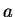and every integer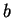,is positive andis negative.

2. There are integersandso thatis positive andis negative.

3. For every integer, there is an integerso thatis positive andis negative.

4. There is an integerso that, for every integer,is positive andis negative.

3. Consider the following symbolic description of kinship''. Our domain is a set of people, and we have the predicates
• m(x) means x is male''.
• f(x) means x is female''.
• P(x,y) means x is the parent of y''.
We have two axioms:
(K1)(K2)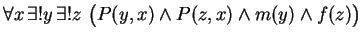1. State carefully, in common English, the meaning of axiom K1.
2. State carefully, in common English, the meaning of axiom K2.
3. Define the predicateto mean. What is the common English meaning of?
4. What is the meaning, in common English, of the assertion?
5. Prove that.

4. Prove that that for any natural number,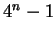is divisible by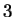. (Hint: use induction on.)

Scott Sutherland 2002-10-11